##### The Japanese Bonsai specialist
Direct order Contact Help / Services Newsletter# Mini bonsai juniperus rigida handbook n°8

Shinpaku mini bonsai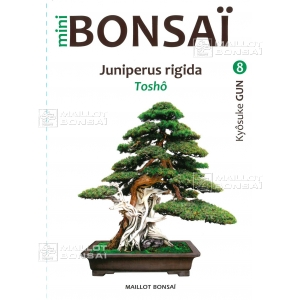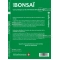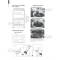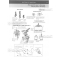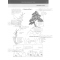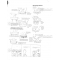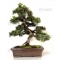ref. : 9521

24,00

Available quantity : 43Order

###### Description

ISBN: 9782953428278

Author: Mr Kyosuke GUN

Format: 137 pages, 17*25 cm

Manga style (read from back to front)

Translated from Japanese into French

This practical 'how to' bonsai book tells you all you need to know about Juniperus rigida. There is currently no English version but easy to understand, clear diagrams and pictures demonstrate how to care for your juniper bonsai throughout the seasons. There are tips on cutting, creating jin and shari, repotting, root trimming and creating different bonsai forms, such as trees on rocks and cascade. This is one of the few books that shares the secrets of creating bonsai.

#bonsai 6.6 #juniperus 3.9 #creating 3.8 #rigida 3.6 #books 3.5 #handbook 2.8 #there 2.5 #this 2.4 #from 2.4 #mini 2.4

Formule
(( ROUND((CHAR_LENGTH(b.article_nom)-CHAR_LENGTH(REPLACE(b.article_nom, 'bonsai', '')))/LENGTH('bonsai')) + ROUND((CHAR_LENGTH(b.article_description)-CHAR_LENGTH(REPLACE(b.article_description, 'bonsai', '')))/LENGTH('bonsai')) ) * 6.6) + (( ROUND((CHAR_LENGTH(b.article_nom)-CHAR_LENGTH(REPLACE(b.article_nom, 'juniperus', '')))/LENGTH('juniperus')) + ROUND((CHAR_LENGTH(b.article_description)-CHAR_LENGTH(REPLACE(b.article_description, 'juniperus', '')))/LENGTH('juniperus')) ) * 3.9) + (( ROUND((CHAR_LENGTH(b.article_nom)-CHAR_LENGTH(REPLACE(b.article_nom, 'creating', '')))/LENGTH('creating')) + ROUND((CHAR_LENGTH(b.article_description)-CHAR_LENGTH(REPLACE(b.article_description, 'creating', '')))/LENGTH('creating')) ) * 3.8) + (( ROUND((CHAR_LENGTH(b.article_nom)-CHAR_LENGTH(REPLACE(b.article_nom, 'rigida', '')))/LENGTH('rigida')) + ROUND((CHAR_LENGTH(b.article_description)-CHAR_LENGTH(REPLACE(b.article_description, 'rigida', '')))/LENGTH('rigida')) ) * 3.6) + (( ROUND((CHAR_LENGTH(b.article_nom)-CHAR_LENGTH(REPLACE(b.article_nom, 'handbook', '')))/LENGTH('handbook')) + ROUND((CHAR_LENGTH(b.article_description)-CHAR_LENGTH(REPLACE(b.article_description, 'handbook', '')))/LENGTH('handbook')) ) * 2.8) + (( ROUND((CHAR_LENGTH(b.article_nom)-CHAR_LENGTH(REPLACE(b.article_nom, 'there', '')))/LENGTH('there')) + ROUND((CHAR_LENGTH(b.article_description)-CHAR_LENGTH(REPLACE(b.article_description, 'there', '')))/LENGTH('there')) ) * 2.5) + (( ROUND((CHAR_LENGTH(b.article_nom)-CHAR_LENGTH(REPLACE(b.article_nom, 'from', '')))/LENGTH('from')) + ROUND((CHAR_LENGTH(b.article_description)-CHAR_LENGTH(REPLACE(b.article_description, 'from', '')))/LENGTH('from')) ) * 2.4) + (( ROUND((CHAR_LENGTH(b.article_nom)-CHAR_LENGTH(REPLACE(b.article_nom, 'this', '')))/LENGTH('this')) + ROUND((CHAR_LENGTH(b.article_description)-CHAR_LENGTH(REPLACE(b.article_description, 'this', '')))/LENGTH('this')) ) * 2.4) + (( ROUND((CHAR_LENGTH(b.article_nom)-CHAR_LENGTH(REPLACE(b.article_nom, 'mini', '')))/LENGTH('mini')) + ROUND((CHAR_LENGTH(b.article_description)-CHAR_LENGTH(REPLACE(b.article_description, 'mini', '')))/LENGTH('mini')) ) * 2.4) + (( ROUND((CHAR_LENGTH(b.article_nom)-CHAR_LENGTH(REPLACE(b.article_nom, 'demonstrate', '')))/LENGTH('demonstrate')) + ROUND((CHAR_LENGTH(b.article_description)-CHAR_LENGTH(REPLACE(b.article_description, 'demonstrate', '')))/LENGTH('demonstrate')) ) * 2.1)

## Secure payment## Delivery

Our logistic partners :04 74 55 23 48
Pépinière MAILLOT-BONSAÏ
Le Bois Frazy
01990 RELEVANT - FRANCE
on appointment